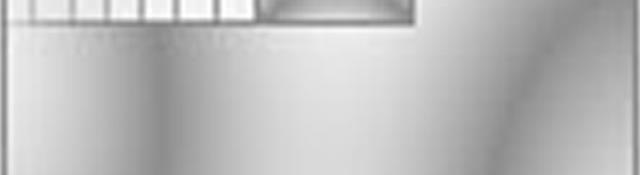## Template matching

Those aren't the templates we're talking about. Template matching is an algorithm that can help you locate certain features in a given image. But the condition is, you need to know exactly what you're looking for.

An example might make it clearer. Lets say you have the image below:

Lets say we're looking for a "p" in the image. So, we're looking for the following image:

Thats' the "template" we're looking for in the original image. I hope that takes care of the template part of the title. Now for the matching part...

## Matching

Template matching works by "sliding" the template across the original image. As it slides, it compares or matches the template to the portion of the image directly under it.

It does this matching by calculating a number. This number denotes the extent to which the template and the portion of the original are equal. The actual number depends on the calculation used. Some denote a complete match by a 0 (indicating no difference between the template and the portion of original) or a 1 (indicating a complete match).

## How OpenCV does template matching

When you perform template matching in OpenCV, you get an image that shows the degree of "equality" or correlation between the template and the portion under the template.

The template is compared against its background, and the result of the calculation (a number) is stored at the top left pixel. Here's what the actual "correlation map" looks like:

The greater the intensity, the greater the correlation between the template and the portion. As you can see, the two "t" in the side in the original image don't really match with the p, so you get a relatively darker region there.

To find out where the P lies in the image, you simply find the location where you have the greatest correlation (or, minimum difference) and that would be your answer.

## On with the code

Enough of theory, now we begin with the code. Create a new Win32 console project, name it whatever you want and accept the default settings. You'll get an empty project with a main function. First, add these header files to the code:

```#include "cv.h"
#include "highgui.h"
```

Next, add the library files to the project. Go to Project > Properties > Configuration > Linker > Input and write cv.lib cxcore.lib cvaux.lib highgui.lib in Additional Dependencies.

If you have any problems with setting this up, I suggest you go through Installing and Getting OpenCV running and "Hello, world!" with images!

Now we get into the main function:

```int main()
{
```

Next, we load the original image and the template:

```    IplImage* imgOriginal = cvLoadImage("technophilia.jpg", 0);
```

Note that I force loading as grayscale image, just to keep things simple. Next, we create a blank image the will hold the correlation map:

```    IplImage* imgResult = cvCreateImage(cvSize(imgOriginal->width-imgTemplate->width+1, imgOriginal->height-imgTemplate->height+1), IPL_DEPTH_32F, 1);
cvZero(imgResult);
```

See the weird size we're giving this image? That has a perfectly sane explanation. Have a look at the picture below:

The correlation map can only extend from the top left corner to the big black dot on the lower right corner. Thats because if the template were to slide any further, you'd get a partial template image... and that would be absurd. So you subtract the height and width of the template from the original image's height and width, and add one.

Now for the instruction-of-the-tutorial:

```    cvMatchTemplate(imgOriginal, imgTemplate, imgResult, CV_TM_CCORR_NORMED);
```

This instruction does all the sliding and correlation mathematics using imgOriginal (the source), imgTemplate (the template) and puts the correlation map into imgResult. The calculations used for determining the correlation map is the last parameter, CV_TM_CCORR_NORMED.

OpenCV offers six different calculation methods:

• CV_TM_SQDIFF
• CV_TM_SQDIFF_NORMED
• CV_TM_CCORR
• CV_TM_CCORR_NORMED
• CV_TM_CCOEFF
• CV_TM_CCOEFF_NORMED

The NORMED calculations give values upto 1.0... the other ones return huge values. SQDIFF is a difference based calculation that gives a 0 at a perfect match. The other two (CCORR and CCOEFF) are correlation based, and return a 1.0 for a perfect match.

To determine the maximum point in the correlation, we use another OpenCV function: cvMinMaxLoc. This function returns the minimum and maximum values and their locations. So, we use it:

```    double min_val=0, max_val=0;
CvPoint min_loc, max_loc;
cvMinMaxLoc(imgResult, &min_val, &max_val, &min_loc, &max_loc);
```

Now max_loc holds the point we're interested in: the point with maximum correlation. We'll just put a rectangle there, and also print out the actual value of correlation... just for the sake of demonstration:

```    cvRectangle(imgOriginal, max_loc, cvPoint(max_loc.x+imgTemplate->width, max_loc.y+imgTemplate->height), cvScalar(0), 1);
printf("%f", max_val);
```

And we finally display the modified original image, and exit:

```    cvNamedWindow("result");
cvShowImage("result", imgOriginal);
cvWaitKey(0);
return 0;

}
```

Here's the result I got:

That seems very accurate. I got a correlation of 0.98. That's because of the little R. It isn't present in the template, so it takes its toll. That, and the fact that JPEG images put a little noise around the edges, combined reduce the correlation.

Well, the first disadvantage is that you need to know what you're looking for. If you're looking for dynamic features, you'll be better off using some other techniques.

Secondly, template matching provided by OpenCV doesn't let you check for rotations and scalings. If the P in our example was rotated by 90 degrees, the current program would never find it. You could write code for it though. A brute force algorithm would be to generate all possible rotations, all possible scales and then do the matching. But that would be extremely slow. So again, use some other techniques.

## We're done!

Hope you learned something from this tutorial. I tried to be as clear and precise as I could.

#### Related posts#### 7 unique neural network architectures